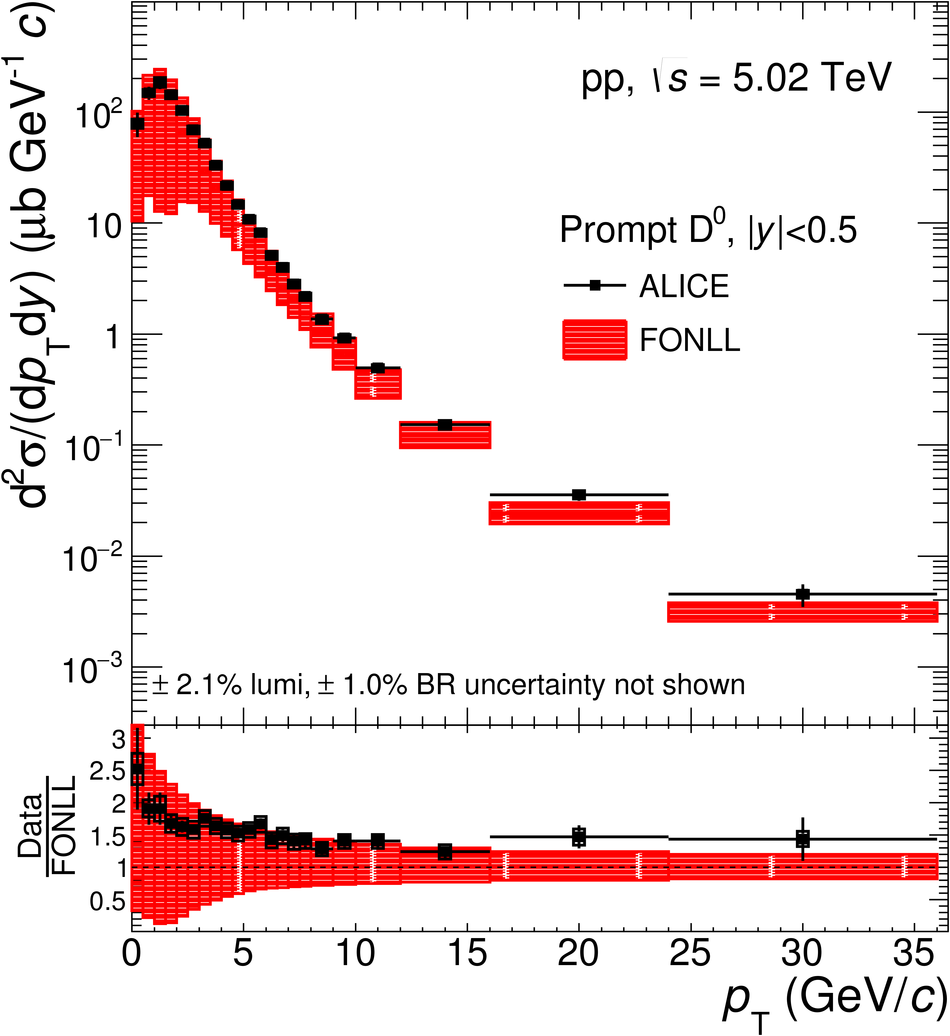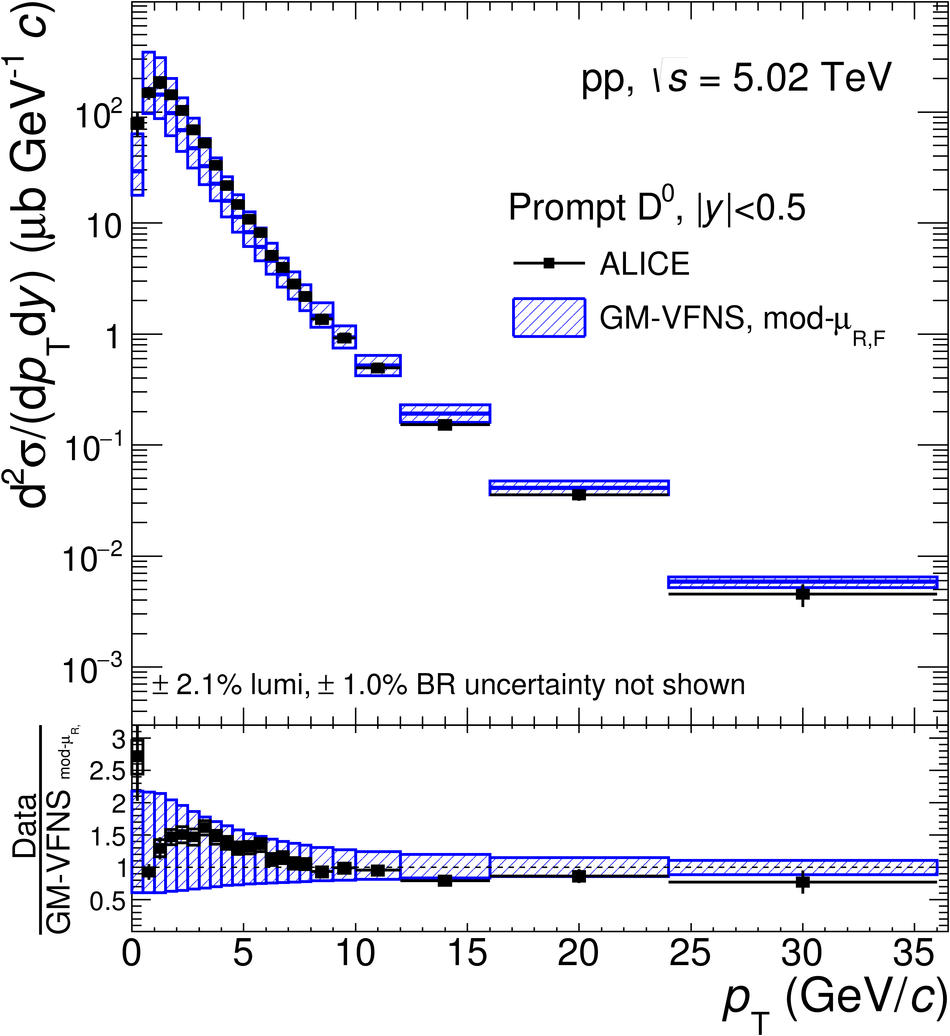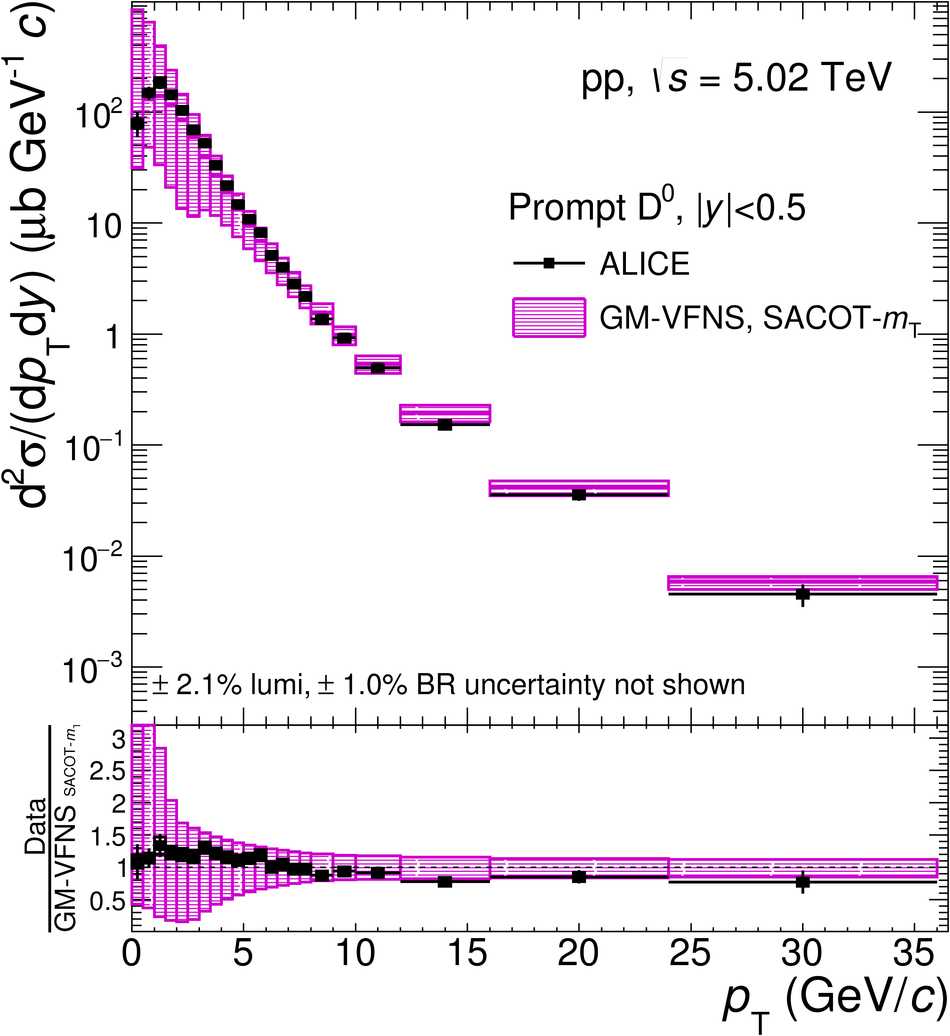# Figure 8

 $\pt$-differential production cross sections for prompt $\Dzero$ meson compared to pQCD calculations: FONLL~, GM-VFNS(mod-$\mu_{\rm R,F}$)~, GM-VFNS(SACOT-$m_{\rm T}$)~, and $k_{\rm T}$-factorisation~. The ratios of the data to the theoretical predictions are shown in the lower part of each panel.